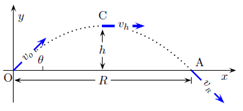Problem: A particle of mass m near the surface of Earth is launched with an initial velocity v0 at an angle θ above the horizontal. Using the origin as the pivot, find the angular momentum when the particle is at the highest point of its trajectory. The z-axis points out of the page. 1. L = mv03/2g sinθ cos2 θ k 2. L = mv03/2g sin2 θ cosθ k 3. L = mv02/2g sin2 θ cosθ k 4. L = - mv02/2g sinθ cos2 θ k 5. L = - mv03/2g sin2 θ cosθ k 6. L = mv02/2g sinθ cosθ k 7. L = - mv02/2g sin2 θ cosθ k 8. L = - mv02/2g tan3 θ k 9. L = mv02/2g tan3 θ k 10. L = - mv02/2g sin θ cos2 θ k

FREE Expert Solution
80% (136 ratings)
Problem Details

A particle of mass m near the surface of Earth is launched with an initial velocity v0 at an angle θ above the horizontal. Using the origin as the pivot, find the angular momentum when the particle is at the highest point of its trajectory. The z-axis points out of the page.

1. = mv03/2g sinθ cos2 θ k

2. = mv03/2g sin2 θ cosθ k

3. = mv02/2g sin2 θ cosθ k

4. = - mv02/2g sinθ cos2 θ k

5. = - mv03/2g sin2 θ cosθ k

6. = mv02/2g sinθ cosθ k

7. = - mv02/2g sin2 θ cosθ k

8. = - mv02/2g tan3 θ k

9. = mv02/2g tan3 θ k

10. = - mv02/2g sin θ cosθ k